Share

# Balbharati solutions for Textbook for SSC Class 10 Mathematics 1 chapter 2 - Quadratic Equations [Latest edition]

Textbook page

#### Chapters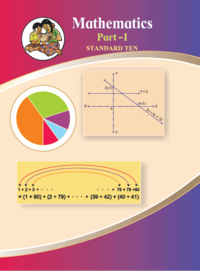Practice Set 2.1OthersPractice Set 2.2Practice Set 2.3Practice Set 2.4Practice Set 2.5Practice Set 2.6Problem Set - 2

#### Balbharati solutions for Textbook for SSC Class 10 Mathematics 1 Chapter 2 Quadratic Equations Exercise Practice Set 2.1 [Page 34]

Practice Set 2.1 | Q 1 | Page 34

Practice Set 2.1 | Q 2.1 | Page 34

Decide whether the following equation is quadratic equation or not.

x2 + 5x – 2 = 0

Practice Set 2.1 | Q 2.2 | Page 34

Decide whether the following equation is quadratic equation or not.

y= 5y – 10

Practice Set 2.1 | Q 2.3 | Page 34

Decide whether the following equation is quadratic equation or not.

$y^2 + \frac{1}{y} = 2$

Practice Set 2.1 | Q 2.4 | Page 34

Decide whether the following equation is quadratic equation or not.

$x + \frac{1}{x} = - 2$

Practice Set 2.1 | Q 2.5 | Page 34

Decide whether the following equation is quadratic equation or not.

(m + 2) (m – 5) = 0

Practice Set 2.1 | Q 2.6 | Page 34

Decide whether the following equation is quadratic equation or not.

m+ 3m2 – 2 = 3m3

Practice Set 2.1 | Q 3.1 | Page 34

Write the following equation in the form ax2 + bx + c= 0, then write the values of a, b, c for the equation.

2y = 10 – y2

Practice Set 2.1 | Q 3.2 | Page 34

Write the following equation in the form ax2 + bx + c= 0, then write the values of a, b, c for the equation.

(x – 1)2 = 2x + 3

Practice Set 2.1 | Q 3.3 | Page 34

Write the following equation in the form ax2 + bx + c= 0, then write the values of a, b, c for the equation.

x2 + 5x = –(3 – x)

Practice Set 2.1 | Q 3.4 | Page 34

Write the following equation in the form ax2 + bx + c= 0, then write the values of a, b, c for the equation.

3m2 = 2m2 – 9

Q 3.5 | Page 34

Write the following equation in the form ax2 + bx + c= 0, then write the values of a, b, c for equation.

P(3+6p) = –5

Practice Set 2.1 | Q 3.6 | Page 34

Write the following equation in the form ax2 + bx + c= 0, then write the values of a, b, c for the equation.

x2 – 9 = 13

Practice Set 2.1 | Q 4.1 | Page 34

Determine whether the values given against the quadratic equation are the roots of the equation.

x2 + 4x – 5 = 0 , x = 1, –1

Practice Set 2.1 | Q 4.2 | Page 34

Determine whether the values given against the quadratic equation are the roots of the equation.

2m2 – 5m = 0, m = 2, 5/2

Practice Set 2.1 | Q 5 | Page 34

Find k if x = 3 is a root of equation kx2 – 10x + 3 = 0

Practice Set 2.1 | Q 6 | Page 34

One of the roots of equation 5m2 + 2m + k = 0 is $\frac{- 7}{5}$ Complete the following activity to find the value of 'k'.

#### Balbharati solutions for Textbook for SSC Class 10 Mathematics 1 Chapter 2 Quadratic Equations Exercise Practice Set 2.2 [Pages 36 - 37]

Practice Set 2.2 | Q 1.01 | Page 36

Solve the following quadratic equation by factorisation.

x2 – 15x + 54 = 0

Practice Set 2.2 | Q 1.02 | Page 36

Solve the following quadratic equation by factorisation.

x2 + x – 20 = 0

Practice Set 2.2 | Q 1.03 | Page 36

Solve the following quadratic equation by factorisation.

2y2 + 27y + 13 = 0

Practice Set 2.2 | Q 1.04 | Page 36

Solve the following quadratic equation by factorisation.

5m2 = 22m + 15

Practice Set 2.2 | Q 1.05 | Page 36

Solve the following quadratic equation by factorization.

2x2 – 2x +$\frac{1}{2}$=0.

Practice Set 2.2 | Q 1.06 | Page 36

Solve the following quadratic equation by factorisation.

$6x - \frac{2}{x} = 1$

Practice Set 2.2 | Q 1.07 | Page 36

Solve the following quadratic equation by factorisation.

$\sqrt{2} x^2 + 7x + 5\sqrt{2} = 0$  to solve this quadratic equation by factorisation, complete the following activity.

√2 x2 + 7 x + 5 √2 = 0
√2 x2 +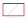++ 5 √2 = 0
x(. . . . .) + √2 (. . . . .) = 0

(. . . . .)(x + 2 ) = 0
(. . . . .) = 0 or (x + 2 ) = 0
∴ x =or x = - 2and - √2 are roots of the equation.

Practice Set 2.2 | Q 1.08 | Page 37

Solve the following quadratic equation by factorisation.

$3 x^2 - 2\sqrt{6}x + 2 = 0$

Practice Set 2.2 | Q 1.09 | Page 37

Solve the following quadratic equation by factorisation.

$2m\left( m - 24 \right) = 50$

Practice Set 2.2 | Q 1.1 | Page 37

Solve the following quadratic equation by factorisation.

$25 m^2 = 9$

Practice Set 2.2 | Q 1.11 | Page 37

Solve the following quadratic equation by factorisation.

7m2 = 21m

Practice Set 2.2 | Q 1.12 | Page 37

Solve the following quadratic equation by factorisation.

$m^2 - 11 = 0$

#### Balbharati solutions for Textbook for SSC Class 10 Mathematics 1 Chapter 2 Quadratic Equations Exercise Practice Set 2.3 [Page 39]

Practice Set 2.3 | Q 1.1 | Page 39

Solve the following quadratic equation by completing the square method.

x2 + x – 20 = 0

Practice Set 2.3 | Q 1.2 | Page 39

Solve the following quadratic equation by completing the square method.

x2 + 2x – 5 = 0

Practice Set 2.3 | Q 1.3 | Page 39

Solve the following quadratic equation by completing the square method.

m2 – 5m = –3

Practice Set 2.3 | Q 1.4 | Page 39

Solve the following quadratic equation by completing the square method.

9y2 – 12y + 2 = 0

Practice Set 2.3 | Q 1.5 | Page 39

Solve the following quadratic equation by completing the square method.

2y2 + 9y +10 = 0

Practice Set 2.3 | Q 1.6 | Page 39

Solve the following quadratic equation by completing the square method.

5x2 = 4x + 7

#### Balbharati solutions for Textbook for SSC Class 10 Mathematics 1 Chapter 2 Quadratic Equations Exercise Practice Set 2.4 [Pages 43 - 44]

Practice Set 2.4 | Q 1.1 | Page 43

Compare the given quadratic equation to the general form and write values of a,b, c.

x2 – 7x + 5 = 0

Practice Set 2.4 | Q 1.2 | Page 43

Compare the given quadratic equation to the general form and write values of a,b, c.

2m2 = 5m – 5

Practice Set 2.4 | Q 1.3 | Page 43

Compare the given quadratic equation to the general form and write values of a,b, c.

y2 = 7y

Practice Set 2.4 | Q 2.1 | Page 43

Solve using formula.

x2 + 6x + 5 = 0

Practice Set 2.4 | Q 2.2 | Page 43

Solve using formula.

x2 – 3x – 2 = 0

Practice Set 2.4 | Q 2.3 | Page 43

Solve using formula.

3m2 + 2m – 7 = 0

Practice Set 2.4 | Q 2.4 | Page 43

Solve using formula.

5m2 – 4m – 2 = 0

Practice Set 2.4 | Q 2.5 | Page 43

Solve using formula.

$y^2 + \frac{1}{3}y = 2$

Practice Set 2.4 | Q 2.6 | Page 43

Solve using formula.

5x2 + 13x + 8 = 0

Practice Set 2.4 | Q 3 | Page 44

With the help of the flow chart given below solve the equation $x^2 + 2\sqrt{3}x + 3 = 0$ using the formula.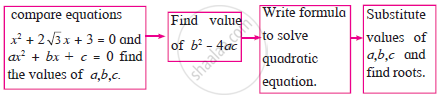#### Balbharati solutions for Textbook for SSC Class 10 Mathematics 1 Chapter 2 Quadratic Equations Exercise Practice Set 2.5 [Pages 49 - 50]

Practice Set 2.5 | Q 1.1 | Page 49

Fill in the gaps and complete.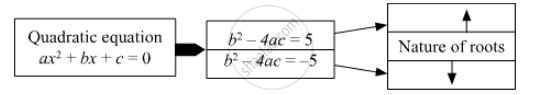Practice Set 2.5 | Q 1.2 | Page 49

Fill in the gap and complete.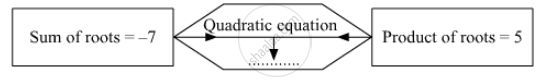Practice Set 2.5 | Q 1.3 | Page 49

Fill in the gaps and complete.

If α, β are roots of quadratic equation,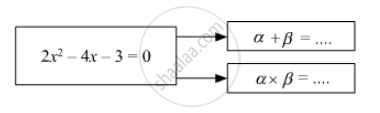Practice Set 2.5 | Q 2.1 | Page 49

Find the value of discriminant.

x2 + 7x – 1 = 0

Practice Set 2.5 | Q 2.2 | Page 49

Find the value of discriminant.

2y2 – 5y + 10 = 0

Practice Set 2.5 | Q 2.3 | Page 49

Find the value of discriminant.

$\sqrt{2} x^2 + 4x + 2\sqrt{2} = 0$

Practice Set 2.5 | Q 3.1 | Page 49

Determine the nature of roots of the following quadratic equation.

x2 – 4x + 4 = 0

Practice Set 2.5 | Q 3.2 | Page 49

Determine the nature of roots of the following quadratic equation.

2y– 7y +2 = 0

Practice Set 2.5 | Q 3.3 | Page 49

Determine the nature of roots of the following quadratic equation.

m2 + 2m + 9 = 0

Practice Set 2.5 | Q 4.1 | Page 50

Form the quadratic equation from the roots given below.

0 and 4

Practice Set 2.5 | Q 4.2 | Page 50

Form the quadratic equation from the roots given below.

3 and –10

Practice Set 2.5 | Q 4.3 | Page 50

Form the quadratic equation from the roots given below.

$\frac{1}{2}, - \frac{1}{2}$

Practice Set 2.5 | Q 4.4 | Page 50

Form the quadratic equation from the roots given below.

$2 - \sqrt{5}, 2 + \sqrt{5}$

Practice Set 2.5 | Q 5 | Page 50

Sum of the roots of a quadratic equation is double their product. Find k if equation x2 – 4kx + k + 3 = 0

Practice Set 2.5 | Q 6.1 | Page 50

α, β are roots of y2 – 2y –7 = 0 find,

α2 + β

Practice Set 2.5 | Q 6.2 | Page 50

α, β are roots of y2 – 2y –7 = 0 find,

α3 + β

Practice Set 2.5 | Q 7.1 | Page 50

The roots of the following quadratic equation is real and equal, find k.

3y+ ky +12 = 0

Practice Set 2.5 | Q 7.2 | Page 50

The roots of the following quadratic equation is real and equal, find k.

kx (x – 2) + 6 = 0

#### Balbharati solutions for Textbook for SSC Class 10 Mathematics 1 Chapter 2 Quadratic Equations Exercise Practice Set 2.6 [Page 52]

Practice Set 2.6 | Q 1 | Page 52

Product of Pragati’s age 2 years ago and 3 years hence is 84. Find her present age.

Practice Set 2.6 | Q 2 | Page 52

The sum of squares of two consecutive natural numbers is 244; find the numbers.

Practice Set 2.6 | Q 3 | Page 52

In the orange garden of Mr. Madhusudan there are 150 orange trees. The number of trees in each row is 5 more than that in each column. Find the number of trees in each row and each column with the help of following flow chart.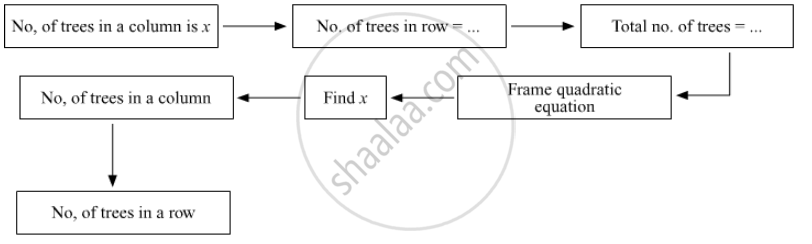Practice Set 2.6 | Q 4 | Page 52

Vivek is older than Kishor by 5 years. The sum of the reciprocals of their ages is $\frac{1}{6}$ Find their present ages.

Practice Set 2.6 | Q 5 | Page 52

Suyash scored 10 marks more in second test than that in the first. 5 times the score of the second test is the same as square of the score in the first test. Find his score in the first test.

Practice Set 2.6 | Q 6 | Page 52

Mr. Kasam runs a small business of making earthen pots. He makes certain number of pots on daily basis. Production cost of each pot is Rs 40 more than 10 times total number of pots, he makes in one day. If production cost of all pots per day is Rs 600, find production cost of one pot and number of pots he makes per day.

Practice Set 2.6 | Q 7 | Page 52

Pratik takes 8 hours to travel 36 km down stream and return to the same spot. The speed of boat in still water is 12 km. per hour. Find the speed of water current.

Practice Set 2.6 | Q 8 | Page 52

Pintu takes 6 days more than those of Nishu to complete certain work. If they work together they finish it in 4 days. How many days would it take to complete the work if they work alone.

Practice Set 2.6 | Q 9 | Page 52

If 460 is divided by a natural number, quotient is 6 more than five times the divisor and remainder is 1. Find quotient and diviser.

Practice Set 2.6 | Q 10 | Page 52

In the adjoining fig. $\square$ ABCD is a trapezium AB || CD and its area is 33 cm2 . From the information given in the figure find the lengths of all sides of the $\square$ABCD. Fill in the empty boxes to get the solution.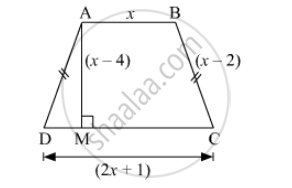#### Balbharati solutions for Textbook for SSC Class 10 Mathematics 1 Chapter 2 Quadratic Equations Exercise Problem Set - 2 [Pages 53 - 54]

Problem Set - 2 | Q 1.1 | Page 53

Choose the correct answer for the following question.
Which one is the quadratic equation?

• $\frac{5}{x} - 3 = x^2$

• x ( x+5 ) = 2

• n - 1 = 2n

• 1/x^2(x+2)=x

Problem Set - 2 | Q 1.2 | Page 53

Choose the correct answer for the following question.
Out of the following equations which one is not a quadratic equation?

• x^2+4x=11+x^2

• x^2=4x

• 5x^2=90+5

• 2x-x^2=x^2

Problem Set - 2 | Q 1.3 | Page 53

Choose the correct answer for the following question.

The roots of x2 + kx + k = 0 are real and equal, find k.

• 0

• 4

• 0 or 4

• 2

Problem Set - 2 | Q 1.4 | Page 53

Choose the correct answer for the following question.
For $\sqrt{2} x^2 - 5x + \sqrt{2} = 0$ find the value of the discriminant.

• -5

• 17

• sqrt2

• 2sqrt2-5

Problem Set - 2 | Q 1.5 | Page 53

Choose the correct answer for the following question.
Which of the following quadratic equations has roots 3,5?

• x2- 15x + 8 = 0

• x2 - 8x + 15 = 0

• x2 + 3x + 5 = 0

• x2 + 8x - 15 = 0

Problem Set - 2 | Q 1.6 | Page 53

Choose the correct answer for the following question.

Out of the following equations, find the equation having the sum of its roots –5.

• 3x2 - 15x + 3 = 0

• x2 - 5x + 3 = 0

• x2 + 3x - 5 = 0

• 3x2 + 15x + 3 = 0

Problem Set - 2 | Q 1.7 | Page 53

Choose the correct answer for the following question.

$\sqrt{5} m^2 - \sqrt{5}m + \sqrt{5} = 0$ which of the following statement is true for this given equation?

• Real and uneual roots

• Real and equal roots

• Roots are not real

• Three roots

Problem Set - 2 | Q 1.8 | Page 53

Choose the correct answer for the following question.

One of the roots of equation x2 + mx – 5 = 0 is 2; find m.

• -2

• -1/2

• 1/2

• 2

Problem Set - 2 | Q 2.1 | Page 54

x2 + 2x + 11 = 0

Problem Set - 2 | Q 2.2 | Page 54

$x^2 - 2x + 5 = x^2$

Problem Set - 2 | Q 2.3 | Page 54

$\left( x + 2 \right)^2 = 2 x^2$

Problem Set - 2 | Q 3.1 | Page 54

Find the value of discriminant of the following equation.

$2 y^2 - y + 2 = 0$

Problem Set - 2 | Q 3.2 | Page 54

Find the value of discriminant of the following equation.

5m2 - m = 0

Problem Set - 2 | Q 3.3 | Page 54

Find the value of discriminant of the following equation.

$\sqrt{5} x^2 - x - \sqrt{5} = 0$

Problem Set - 2 | Q 4 | Page 54

One of the roots of quadratic equation $2 x^2 + kx - 2 = 0$ is –2. find k.

Problem Set - 2 | Q 5.1 | Page 54

Two roots of quadratic equation is given ; frame the equation.

10 and –10

Problem Set - 2 | Q 5.2 | Page 54

Two roots of quadratic equation is given ; frame the equation.

$1 - 3\sqrt{5} \text{ and } 1 + 3\sqrt{5}$

Problem Set - 2 | Q 5.3 | Page 54

Two roots of quadratic equation is given ; frame the equation.

0 and 7

Problem Set - 2 | Q 6.1 | Page 54

Determine the nature of root of the quadratic equation.

$3 x^2 - 5x + 7 = 0$

Problem Set - 2 | Q 6.2 | Page 54

Determine the nature of root of the quadratic equation.

$\sqrt{3} x^2 + \sqrt{2}x - 2\sqrt{3} = 0$

Problem Set - 2 | Q 6.3 | Page 54

Determine the nature of root of the quadratic equation.

m2 - 2m + 1 = 0

Problem Set - 2 | Q 7.1 | Page 54

$\frac{1}{x + 5} = \frac{1}{x^2}$

Problem Set - 2 | Q 7.2 | Page 54

$x^2 - \frac{3x}{10} - \frac{1}{10} = 0$

Problem Set - 2 | Q 7.3 | Page 54

$\left( 2x + 3 \right)^2 = 25$

Problem Set - 2 | Q 7.4 | Page 54

m2 + 5m + 5 = 0

Problem Set - 2 | Q 7.5 | Page 54

5m2 + 2m + 1 = 0

Problem Set - 2 | Q 7.6 | Page 54

$x^2 - 4x - 3 = 0$

Problem Set - 2 | Q 8 | Page 54

Find m if (m – 12) x2 + 2(m –12) x + 2 = 0 has real and equal roots.

Problem Set - 2 | Q 9 | Page 54

The sum of two roots of a quadratic equation is 5 and sum of their cubes is 35, find the equation.

Problem Set - 2 | Q 10 | Page 54

Find quadratic equation such that its roots are square of sum of the roots and square of difference of the roots of equation

$2 x^2 + 2\left( p + q \right)x + p^2 + q^2 = 0$

Problem Set - 2 | Q 11 | Page 54
Mukund possesses Rs 50 more than what Sagar possesses. The product of the amount they have is 15,000. Find the amount each one has.

Problem Set - 2 | Q 12 | Page 54

The difference between squares of two numbers is 120. The square of smaller number is twice the greater number. Find the numbers.

Problem Set - 2 | Q 13 | Page 54

Ranjana wants to distribute 540 oranges among some students. If 30 students were more each would get 3 oranges less. Find the number of students.

Problem Set - 2 | Q 14 | Page 54

Mr. Dinesh owns an agricultural farm at village Talvel. The length of the farm is 10 meter more than twice the breadth. In order to harvest rain water, he dug a square shaped pond inside the farm. The side of pond is $\frac{1}{3}$ of the breadth of the farm. The area of the farm is 20 times the area of the pond. Find the length and breadth of the farm and of the pond.

Problem Set - 2 | Q 15 | Page 54

A tank fills completely in 2 hours if both the taps are open. If only one of the taps is open at the given time, the smaller tap takes 3 hours more than the larger one to fill the tank. How much time does each tap take to fill the tank completely?

Practice Set 2.1OthersPractice Set 2.2Practice Set 2.3Practice Set 2.4Practice Set 2.5Practice Set 2.6Problem Set - 2## Balbharati solutions for Textbook for SSC Class 10 Mathematics 1 chapter 2 - Quadratic Equations

Balbharati solutions for Textbook for SSC Class 10 Mathematics 1 chapter 2 (Quadratic Equations) include all questions with solution and detail explanation. This will clear students doubts about any question and improve application skills while preparing for board exams. The detailed, step-by-step solutions will help you understand the concepts better and clear your confusions, if any. Shaalaa.com has the Maharashtra State Board Textbook for SSC Class 10 Mathematics 1 solutions in a manner that help students grasp basic concepts better and faster.

Further, we at Shaalaa.com provide such solutions so that students can prepare for written exams. Balbharati textbook solutions can be a core help for self-study and acts as a perfect self-help guidance for students.

Concepts covered in Textbook for SSC Class 10 Mathematics 1 chapter 2 Quadratic Equations are Quadratic Equations Examples and Solutions, Quadratic Equations, Roots of a Quadratic Equation, Nature of Roots, Relation Between Roots of the Equation and Coefficient of the Terms in the Equation Equations Reducible to Quadratic Form, Solutions of Quadratic Equations by Factorization, Solutions of Quadratic Equations by Completing the Square, Formula for Solving a Quadratic Equation.

Using Balbharati Class 10th Board Exam solutions Quadratic Equations exercise by students are an easy way to prepare for the exams, as they involve solutions arranged chapter-wise also page wise. The questions involved in Balbharati Solutions are important questions that can be asked in the final exam. Maximum students of Maharashtra State Board Class 10th Board Exam prefer Balbharati Textbook Solutions to score more in exam.

Get the free view of chapter 2 Quadratic Equations Class 10th Board Exam extra questions for Textbook for SSC Class 10 Mathematics 1 and can use Shaalaa.com to keep it handy for your exam preparation

S